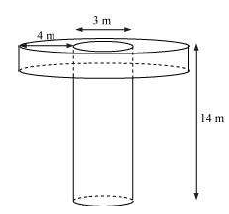Newbie

# A well of diameter 3 m is dug 14 m deep. The earth taken out of it has been spread evenly all around it in the shape of a circular ring of width 4 m to form an embankment. Find the height of the embankment. Q.4

• 0

Find the important question of exercise 13.3 question number 4 of ncert class 10 chapter surface areas and volumes . What is the best solution of this question , also find the simplest and easiest solution of this question .A well of diameter 3 m is dug 14 m deep. The earth taken out of it has been spread evenly all around it in the shape of a circular ring of width 4 m to form an embankment. Find the height of the embankment.

Share

1. The shape of the well will be cylindrical as given below.Given, Depth (h1) of well = 14 m

Diameter of the circular end of the well =3 m

So, Radius (r1) = 3/2 m

Width of the embankment = 4 m

From the figure, it can be said that the embankment will be a cylinder having an outer radius (r2) as 4+(3/2) = 11/2 m and inner radius (r1) as 3/2m

Now, let the height of embankment be h2

∴ Volume of soil dug from well = Volume of earth used to form embankment

π×r12×h = π×(r22-r12) × h2

Solving this, we get,

The height of the embankment (h2) as 1.125 m.

• 0